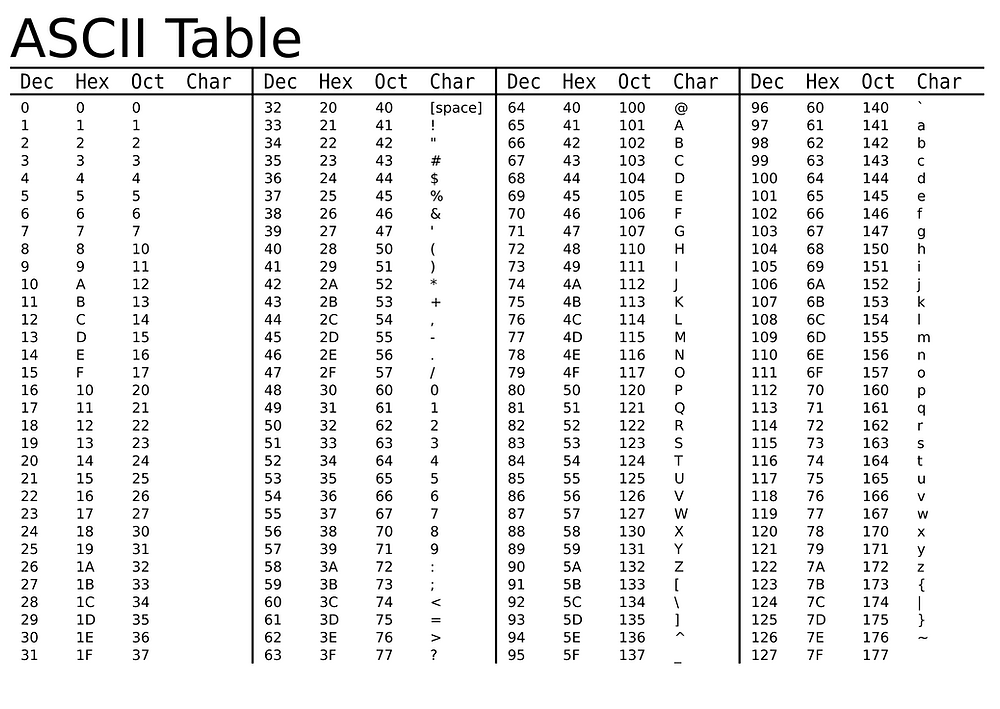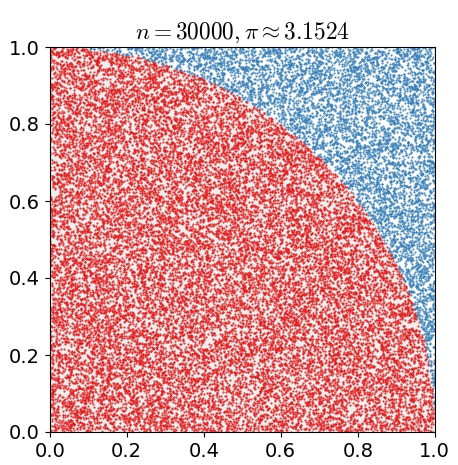•Ting Chun Liu

# Processing 蒙地卡羅演算法做文字動畫## 第一部分：文字流動

for (int x=0; x<=width; x+=10) { for (int y=0; y<=height; y+=10) { text[x][y] = char(int('a'+random(26))); } } for (int x=0; x<=width; x+=10) { for (int y=0; y<=height; y+=10) { fill(255,100); //上色 text(text[x][y], x, y); } }for (int x=0; x<=width; x+=10) { text[x] = (char('a'+int(random(25)))); } for (int y=10; y<=height; y+=10) { for (int x=0; x<=width; x+=10) { if (random(5)>3) text[x][y] = text[x][y-10]; } }

for (int y=height; y>=10; y-=10) for (int y=10; y<height; y+=10)## 第二部分：圖像轉文字char[] index = {'a', 'b', 'c', 'd', 'e', 'f', 'g', 'h', 'i', 'j', 'k', 'l', 'm', 'n', 'o', 'p', 'q', 'r', 's', 't', 'u', 'v', 'w', 'x', 'y', 'z', 'A', 'B', 'C', 'D', 'E', 'F', 'G', 'H', 'I', 'J', 'K', 'L', 'M', 'N', 'O', 'P', 'Q', 'R', 'S', 'T', 'U', 'V', 'W', 'X', 'Y', 'Z', '~', '!', '@', '#', '\$', '%', '^', '&', '*', '(', ')', '-', '+', ' ‘}; float[] index_density;

size(800,800); textAlign(CENTER,CENTER); textSize(500); index_density=new float[index_length];

100萬個點的示意圖。nfc((float(total)/float(1000000))*100, 3));

float[] index_density = {4.595, 5.98, 3.475, 5.964, 4.46, 3.819, 6.538, 5.446, 2.25, 3.421, 5.131, 2.788, 7.01, 4.547, 4.703, 5.808, 5.809, 2.502, 3.456, 3.181,4.626, 3.487, 6.27, 3.945, 4.296, 4.2, 6.145, 7.078, 5.328, 7.283, 5.367, 4.456, 6.068, 6.606, 2.782, 3.793, 5.865, 3.744, 9.116, 7.354, 7.154, 5.516,8.077, 6.755, 4.99, 4.363, 5.898, 4.961, 9.212, 5.624, 4.408, 5.674, 1.737,2.119, 7.047, 5.383, 5.19, 6.321, 2.727, 7.452, 1.506, 2.689, 2.71, 1.173, 2.911, 0.0};

for (int i=0; i<index_length-1; i++) { for (int j=i+1; j<index_length; j++) { if (index_density[i]>index_density[j]) { float tempF = index_density[i]; index_density[i] = index_density[j]; index_density[j] = tempF; char tempC = index[i]; index[i] = index[j]; index[j] = tempC; } } }PImage img = loadImage("XXX.jpg"); for (int x=0; x<width; x+=10) { for (int y=0; y<height; y+=10) { //map對應的畫布位置 int gx = floor(map(x, 0, width, 0, img.width)); int gy = floor(map(y, 0, height, 0, img.height)); fill(255,0,0, 60); text(index[floor(map(brightness(img.get(gx, gy)),255, 0, 0, index_length-1))], x, y); } }https://github.com/aprilcoffee/FontDensityCalculation/blob/master/FontDensityCalculation.pde

353 次查看0 則留言